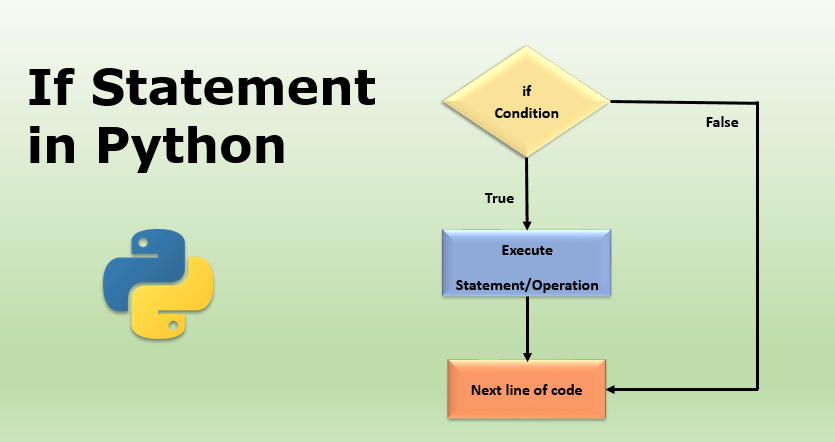# DETAILED BLOG FOR CONDITIONAL STATEMENTS IN PYTHON – 5## OVERVIEW

In this blog, you will learn,

• What are conditional statements?
• Types of conditional statements
• Understanding the types of conditional statements with example.

## What are conditional statements?

In the coding world we have some conditional statements that we use when we have multiple tasks that should be executed based on some conditions. That means if any of the conditions out of all gets true then the task associated with that condition will be executed.

Here is the working flow of the conditional statement.

## Types of conditional statements

Basically there are three types of conditional statements which are:

• if-else
• if-elif-else
• Nested (if-else, if-elif-else)

Now let’s understand about each of them one by one with example in details.

1. if-else Statement

Now, let’s first understand the syntax and working of if-else statements.

``````Syntax:
if <condition>:
<expression>
else:
<expression>``````

Now let’s take an example to understand it. Now we will take one number and will display the output as odd number or even number based on the condition that we’ll put.

```num=5
if num%2==0:
print("Even Number")
else:
print("Odd Number")```
```Output:
Odd Number```

Here, we have an expression num%2 which will return the output as 0 or 1 based on the integer number and here if the remainder is 0 that means the number is even number otherwise it is odd number.

2. if-elif-else

Now, let’s first understand the syntax and working of if-else statements.

``````Syntax:
if <condition>:
<expression>
elif <condition>:
<expression>
else:
<expression>``````

Now let’s take an example to understand it. Now we will take one float number and will display the output as odd number or even number based on the condition that we’ll put.

```num=5.5
if num%2==0:
print("Even Number")
elif num%2==1:
print("Odd Number")
else:
print("It is neither even nor odd number.")```
```Output:
It is neither even nor odd number.```

Now, as per the expression above you can see that we have more than two conditions and more than 2 outputs. The output will be 0, 1 or any decimal value. So as per the condition if the remainder of num%2 is 0 or 1 then the output will be even number or odd. Otherwise, the remainder will be a float value on the basis of which we can say that “It is neither even nor odd number.”

3. Nested Conditional Statements

Now, let’s first understand the syntax and working of if-else statements.

``````Syntax:
if <condition>:
<expression>
if <condition>:
<expression>
elif <condition>:
<expression>
else:
<expression>
elif <condition>:
<expression>
if <condition>:
<expression>
else:
<expression>
else:
<expression>
if <condition>:
<expression>
else:
<expression>``````

For the above expression lets take an example to understand this. Now we’ll take an example where we will ask the user to enter the username and password to login for different cases which are,

• If the username is incorrect then it should not ask for the password and it should print User doesn’t exist
• If the password is incorrect, it should print password is incorrect
``````username = ["Innovate","Ashish"]
uname = input("Enter the user name: ")

paswrd = input("Enter the password: ")
else:
else:
print("User doesn't exist.")``````

Now based on all the condition it will return you the output as shown below,

With the above example problem, I think now you have got a proper understanding of how to use the conditional statements. For more practice here is a problem for you to solve and get a proper understanding of what you have understood till now.

Q 1. Write a program to ask the user to enter the a number to find whether that number is an Adam number or not.

`x=int(input("Enter a number to find adam number or not: "))if int(str(int(int(str(x**2)[::-1])**0.5))[::-1])==x:    print(x,"is an Adam number.")else:    print(x, "is not an Adam number.")`

Check this video more clarification,

Time to wrap up now. Hope you liked our content on comparison statements in python. See you in our next blog, thanks for reading our blog and do leave a comment below to help us improve the content to serve you all of our experience with you. Stay tuned with us for more python programming contents.

Also check out our other playlist Rasa Chatbot, Internet of things, Docker, etc.
Become a member of our social family on youtube here.1.Apneet says:
1.admin says: